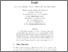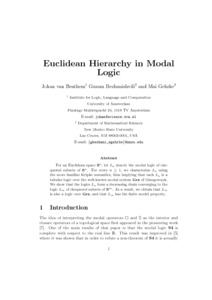# PP-2002-07: Euclidean Hierarchy in Modal Logic

PP-2002-07: van Benthem, Johan and Bezhanishvili, Guram and Gehrke, Mai (2002) Euclidean Hierarchy in Modal Logic. [Report]Preview
Text (Full Text)
PP-2002-07.text.pdfText (Abstract)
PP-2002-07.abstract.txt

For an Euclidean space $\mathbb{R}^n$, let $L_n$ denote the modal logic of chequered subsets of $\mathbb{R}^n$. For every $n\geq 1$, we characterize $L_n$ using the more familiar Kripke semantics, thus implying that each $L_n$ is a tabular logic over the well-known modal system Grz of Grzegorczyk. We show that the logics $L_n$ form a decreasing chain converging to the logic $L_\infty$ of chequered subsets of $\mathbb{R}^\infty$. As a result, we obtain that $L_\infty$ is also a logic over Grz, and that $L_\infty$ has the finite model property.View Item